Courses

# Digital Electronics - MCQ Test - 3

## 25 Questions MCQ Test GATE Electrical Engineering (EE) 2022 Mock Test Series | Digital Electronics - MCQ Test - 3

Description
This mock test of Digital Electronics - MCQ Test - 3 for GATE helps you for every GATE entrance exam. This contains 25 Multiple Choice Questions for GATE Digital Electronics - MCQ Test - 3 (mcq) to study with solutions a complete question bank. The solved questions answers in this Digital Electronics - MCQ Test - 3 quiz give you a good mix of easy questions and tough questions. GATE students definitely take this Digital Electronics - MCQ Test - 3 exercise for a better result in the exam. You can find other Digital Electronics - MCQ Test - 3 extra questions, long questions & short questions for GATE on EduRev as well by searching above.
QUESTION: 1

Solution:
QUESTION: 2

Solution:
QUESTION: 3

###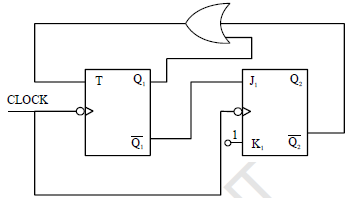The sequence (Q2 Q1) when successive clock pulses are applied

Solution:
QUESTION: 4

A number P represented in signed binary 2’s complement form is 1001101. Another number Q1 represented in signed binary 2’s complement form is 11010111. If Q is subtracted from P, the result expressed in signed binary 2’s complement form

Solution:
QUESTION: 5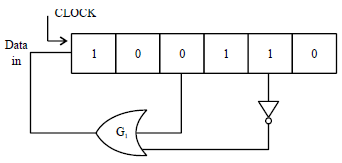Figure shows the initial content of a 6 bit SIPO register with each clock pulse output of gate G1 is pushed in  the left most stage. What will be the content of the register after 9th clock pulse?

Solution:
QUESTION: 6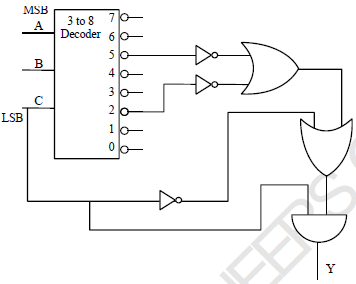The function y (A, B, C) implemented by the circuit shown is

Solution:
QUESTION: 7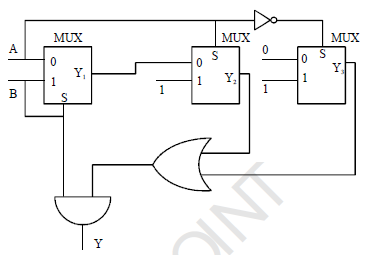The output Y of the circuit shown is

Solution:
QUESTION: 8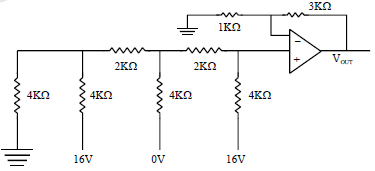The output voltage of the circuit shown

Solution:
QUESTION: 9

In the latch circuit shown if A = 1, B = 0 is applied, the corresponding stable outputs are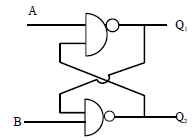Solution:
QUESTION: 10

A digital bit is to be transmitted from point P to point Q by delaying it through a SISO register of 8 bit as shown. If the clock frequency used is 4 MHz, the delay created by the register is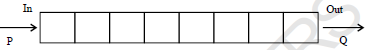Solution:
QUESTION: 11

Identify the incorrect statement

Solution:
QUESTION: 12

The modulus of the circuit (Counter) shown is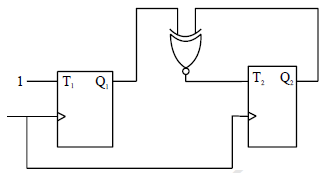Solution:
QUESTION: 13

The resistance corresponding to LSB in a weighted resistor 5 bit DAC i s 64 k?, then the resistor corresponding to MSB is

Solution:
QUESTION: 14

The output of a 6bit DAC for the digital input of 1001000 is 300 volts. What will be the DAC output for digital input 1101010 approximately

Solution:
QUESTION: 15

The simplified expression using kmap in POS form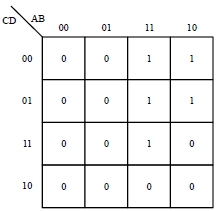Solution:
QUESTION: 16

The modulus of the counter circuit shown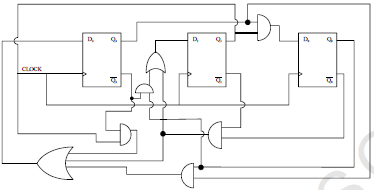Solution:
QUESTION: 17

In the circuit shown in figure, to make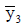Low, what must be conditions at A and B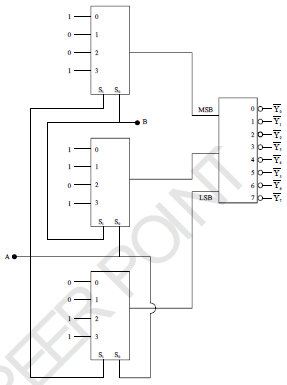Solution:
QUESTION: 18

The number (123)7 in base 5 is equal to

Solution:
QUESTION: 19

The output y of the circuit shown is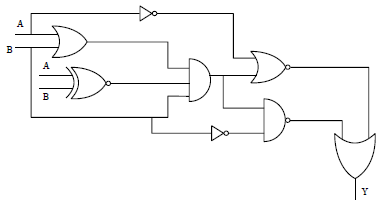Solution:
QUESTION: 20

The counting sequence (Q1 Q2) when clock pulses are applied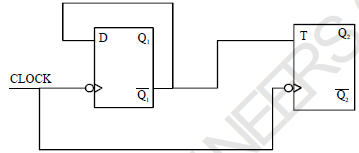Solution:
QUESTION: 21

The binary equivalent of the gray code (1011100 11)G

Solution:
QUESTION: 22

An analog input of 4.20 Volt is to be converted into digital form using counter type ADC. The digital equivalent will be [Taking threshold voltage of comparator as one tenth of DAC resolution)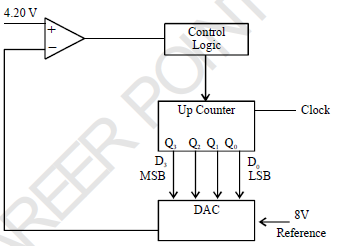Solution:
QUESTION: 23

The present state of a JK flip flop was 0. After one clock pulse, it was found to be 0. What possible inputs
may be applied?

Solution:
QUESTION: 24

Figure below shows a free running oscillation.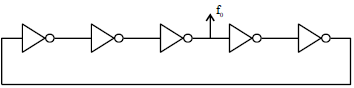Assuming propagation delay of each inverter 10 μs, the output frequency f0 is
(a) (b)
(c) (d)

Solution:
QUESTION: 25

Correct set of universal gates is

Solution: